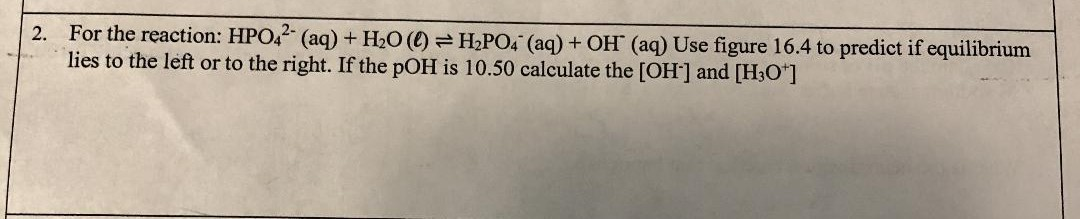# For the reaction: HPO42- (aq) + H2O (l) ⇌ H2PO4- (aq) + OH- (aq) Use figure 16.4 to predict if equilibrium lies to the left or to the right. If the pOH = 10.50, calculate the [OH-] and [H3O+] (predict if equilibrium lies to the left or to the right )